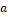## Weekly Overview

Weekly Topics

The focus of this week’s instruction is to deepen students’ understanding of:

• Understanding Subtraction of Integers and Other Rational Numbers
• The Distance Between Two Rational Numbers
• Addition and Subtraction of Rational Numbers
• Understanding Multiplication of Integers
• Division of Integers

Materials Needed

• Equations
• Expressions
• Number Line
• Tape Diagram
• Index Cards
• Tiles
• Personal Whiteboards

Standard(s) Covered

7.NS.A.1 Apply and extend previous understandings of addition and subtraction to add and subtract rational numbers; represent addition and subtraction on a horizontal or vertical number line diagram.

1. Describe situations in which opposite quantities combine to make 0.
2. Understand p + q as the number located a distance |q| from p, in the positive or negative direction depending on whether q is positive or negative. Show that a number and its opposite have a sum of 0 (are additive inverses). Interpret sums of rational numbers by describing real-world contexts.
3. Understand subtraction of rational numbers as adding the additive inverse, p – q = p + (–q). Show that the distance between two rational numbers on the number line is the absolute value of their difference and apply this principle in real-world contexts.
4. Apply properties of operations as strategies to add and subtract rational numbers.

7.NS.A.2 Apply and extend previous understandings of multiplication and division and of fractions to multiply and divide rational numbers.

1. Understand that multiplication is extended from fractions to rational numbers by requiring that operations continue to satisfy the properties of operations, particularly the distributive property, leading to products such as (–1)(–1) = 1 and the rules for multiplying signed numbers. Interpret products of rational numbers by describing real-world contexts.
2. Understand that integers can be divided, provided that the divisor is not zero, and every quotient of integers (with non-zero divisor) is a rational number. If p and q are integers, then –(p/q) = (–p)/q = p/(–q). Interpret quotients of rational numbers by describing real-world contexts.
3. Apply properties of operations as strategies to multiply and divide rational numbers.
4. Convert a rational number to a decimal using long division; know that the decimal form of a rational number terminates in 0s or eventually repeats.

RepresentationsAdditional Terms and Symbols

• Additive Identity (The additive identity is the number 0.)
• Additive Inverse (An additive inverse of a number is a number such that the sum of the two numbers is 0.
• The additive inverse of a number ais the opposite of a (i.e., -a) because if we add both numbers using the vector approach above, we get a+(-a)=0.)
• Formula for the Distance Between Two Numbers (If p and q are numbers on a number line, then the distance between p and q is p-q.)

Familiar Terms

• Absolute Value
• Commutative Property (of Multiplication and Addition)
• Distributive Property (of Multiplication Over Addition)
• Equation
• Expression
• Integer
• Inverse
• Negatives
• Opposites
• Positives
• Rational Numbers